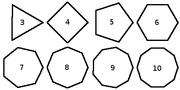11 Pages

Fermat's polygonal number theorem says that every positive number is a sum of n or less n-gonal numbers. It was initially proposed by Pierre de Fermat who produced no proof for it. Gauss later proved the triangular case (that every positive number is a sum of three or more triangular numbers) in 1796.

## N-gonal NumbersN-gonal numbers can be defined in a series. The first part of the series is a triangular number. A triangular number is a 3-gonal number, as it is a number that can be fit into a regular (equilateral) triangle. The next is a 4-gonal number which is a number that can be fit into a regular quadrilateral (square), and next is a 5-gonal number which is a number that can be fit into a regular pentagon, and then a 6-gonal number which is a number that can be fit into a regular hexagon. The theorem for each to determine the xth N-gon is x(3x-1)/2 for a 5-gon, x(4x-2) for a 6-gon, x(5x-3) for a 7-gon and so on. From this, we can determine the xth n-gonal number. The theorem for finding this is {(n^2)(x-2)-(n[x-4])}/2.

Community content is available under CC-BY-SA unless otherwise noted.